# EQUATIONS INEQUALITIES Properties WHAT ARE EQUATIONS Equations are

• Slides: 17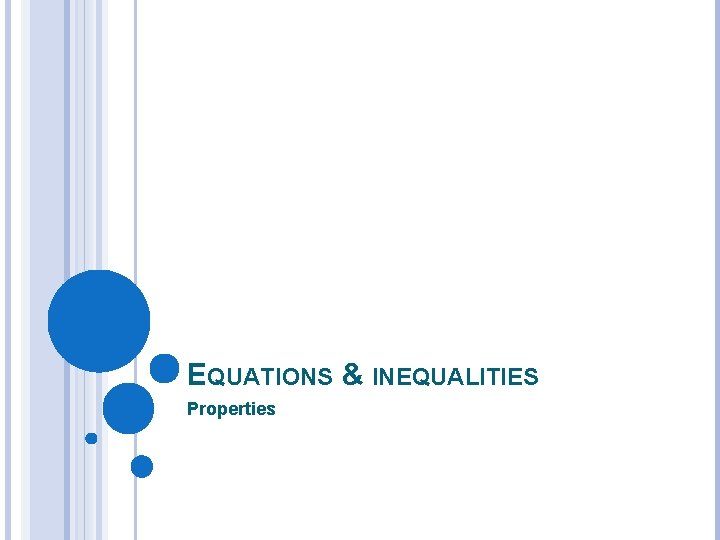EQUATIONS & INEQUALITIES PropertiesWHAT ARE EQUATIONS? Equations are mathematical sentences that state two expressions are equal. Example: 2 x – 5 = 3(x + 4)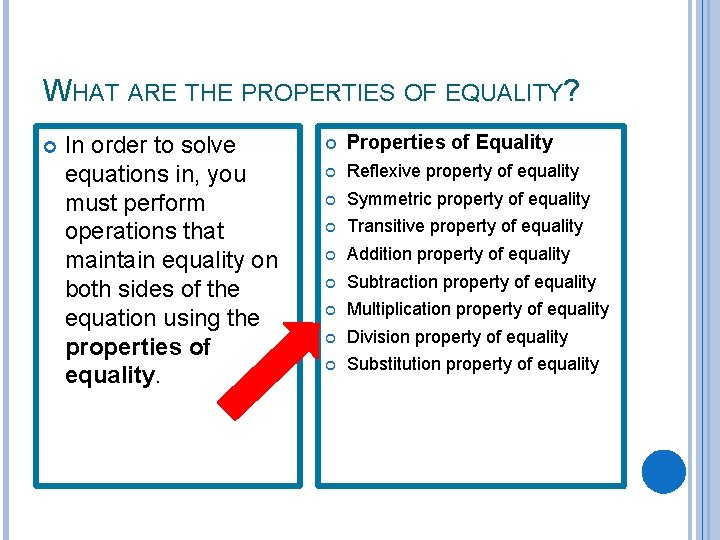WHAT ARE THE PROPERTIES OF EQUALITY? In order to solve equations in, you must perform operations that maintain equality on both sides of the equation using the properties of equality. Properties of Equality Reflexive property of equality Symmetric property of equality Transitive property of equality Addition property of equality Subtraction property of equality Multiplication property of equality Division property of equality Substitution property of equalityPROPERTIES OF EQUALITY Reflexive Property of Equality Symmetric Property of Equality a=a A number is equal to itself. -5 = -5 If a = b, then b = a. If numbers are equal, they will still be equal if the order is changed. If x = 2, then 2 = x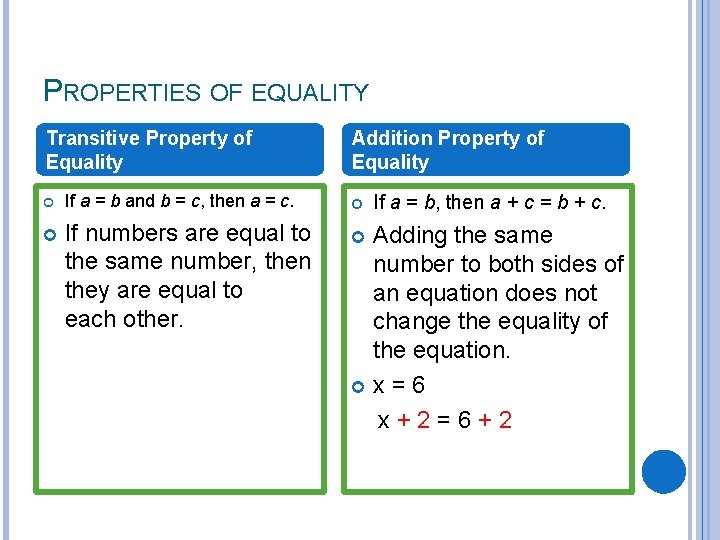PROPERTIES OF EQUALITY Transitive Property of Equality If a = b and b = c, then a = c. If numbers are equal to the same number, then they are equal to each other. Addition Property of Equality If a = b, then a + c = b + c. Adding the same number to both sides of an equation does not change the equality of the equation. x=6 x+2=6+2PROPERTIES OF EQUALITY Subtraction Property of Equality If a = b, then a − c = b − c. Subtracting the same number from both sides of an equation does not change the equality of the equation. x=6 x− 2=6− 2 Multiplication Property of Equality If a = b and c ≠ 0, then a • c = b • c. Multiplying both sides of the equation by the same number, other than 0, does not change the equality of the equation. x=6 x • 2=6 • 2PROPERTIES OF EQUALITY Division Property of Equality If a = b and c ≠ 0, then a ÷ c = b ÷ c. Dividing both sides of the equation by the same number, other than 0, does not change the equality of the equation. If x = 6 then Substitution Property of Equality If a = b, then b may be substituted for a in any expression containing a. If two numbers are equal, then substituting one in for another does not change the equality of the equation.PROPERTIES OF OPERATIONS (REVIEW) Property Rule Example Commutative property of addition a+b=b+a 3+5=5+3 Commutative property of multiplication a • b=b • a 3 • 5=5 • 3 Associative property of addition (a + b) + c = a + (b + c) (3 + 5) + 6 = 3 + (5 + 6) Associative property of multiplication (a • b) • c = a • (b • c) (3 • 5) • 6 = 3 • (5 • 6) Distributive property of multiplication over addition a • (b + c) = a • b + a • c 3 • (5 + 6) = 3 • 5 + 3 • 6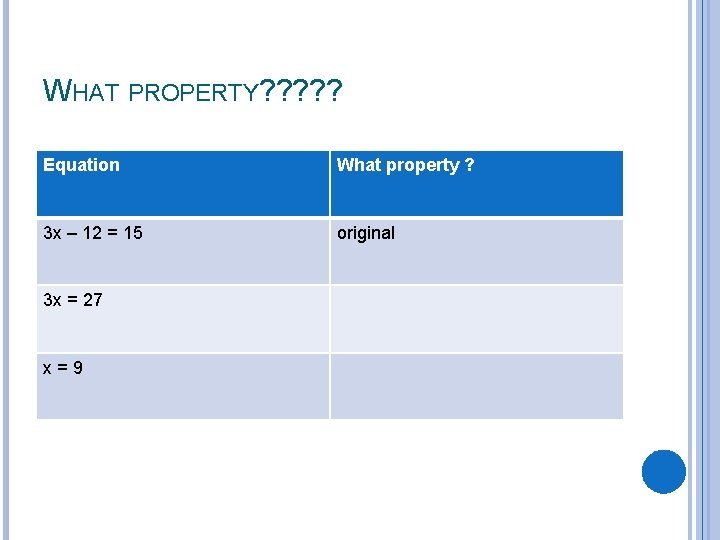WHAT PROPERTY? ? ? Equation What property ? 3 x – 12 = 15 original 3 x = 27 x=9WHAT PROPERTY? ? ? Equation What property ? original x = -18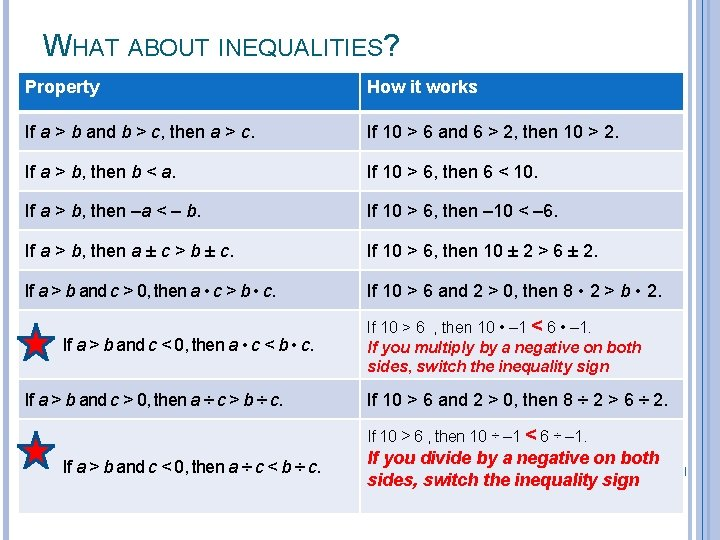WHAT ABOUT INEQUALITIES? Property How it works If a > b and b > c, then a > c. If 10 > 6 and 6 > 2, then 10 > 2. If a > b, then b < a. If 10 > 6, then 6 < 10. If a > b, then –a < – b. If 10 > 6, then – 10 < – 6. If a > b, then a ± c > b ± c. If 10 > 6, then 10 ± 2 > 6 ± 2. If a > b and c > 0, then a • c > b • c. If 10 > 6 and 2 > 0, then 8 • 2 > b • 2. If a > b and c < 0, then a • c < b • c. If a > b and c > 0, then a ÷ c > b ÷ c. If 10 > 6 , then 10 • – 1 < 6 • – 1. If you multiply by a negative on both sides, switch the inequality sign If 10 > 6 and 2 > 0, then 8 ÷ 2 > 6 ÷ 2. If 10 > 6 , then 10 ÷ – 1 < 6 ÷ – 1. If a > b and c < 0, then a ÷ c < b ÷ c. If you divide by a negative on both sides, switch the inequality sign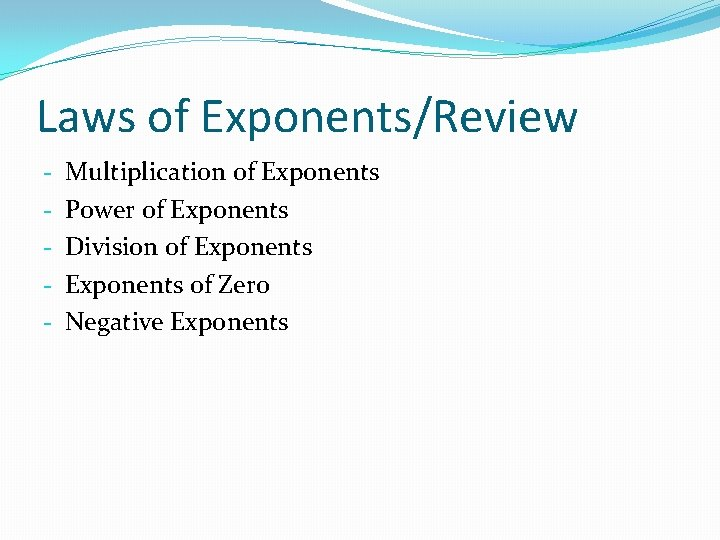Laws of Exponents/Review - Multiplication of Exponents Power of Exponents Division of Exponents of Zero Negative ExponentsMultiplication of Exponents General Rule: Specific Example: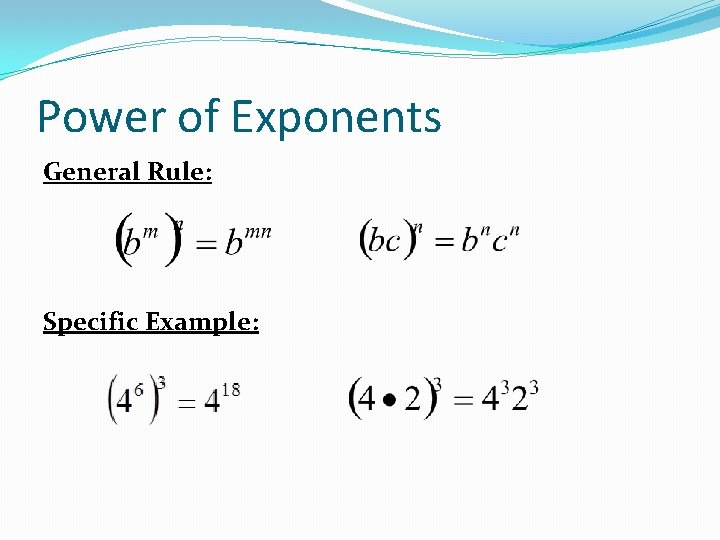Power of Exponents General Rule: Specific Example: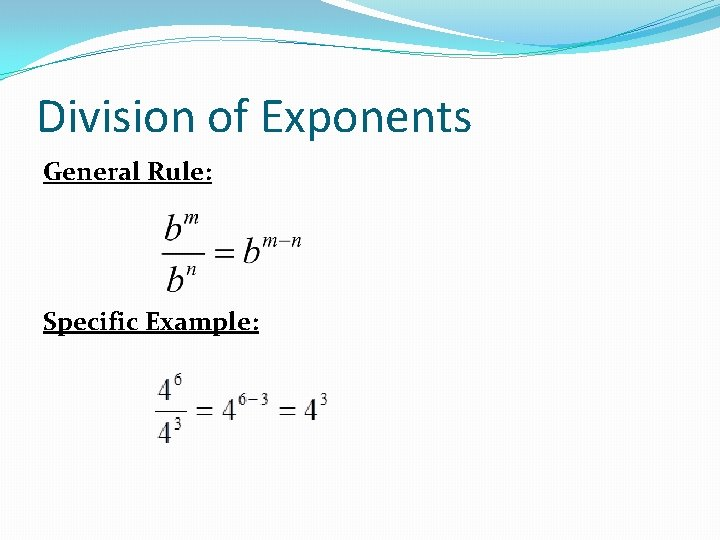Division of Exponents General Rule: Specific Example: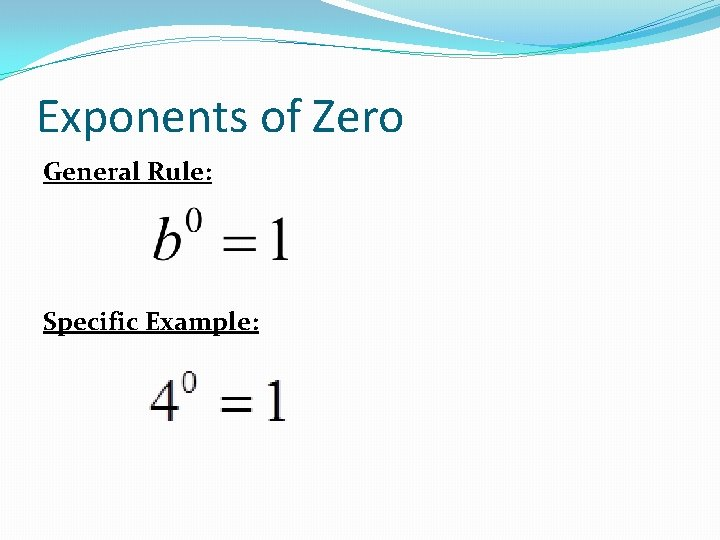Exponents of Zero General Rule: Specific Example:Negative Exponents General Rule: and Specific Example: and